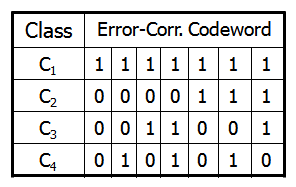# Current Slide

Small screen detected. You are viewing the mobile version of SlideWiki. If you wish to edit slides you will need to use a larger device.

### Error-Correcting Codes for Multiclass Classification

• Originally designed to correct errors during data transmission for communication tasks by exploring data redundancy
• Example
• A 7-bit codeword associated with classes 1-4
• Given a unknown tuple X, the 7-trained classifiers output: 0001010
• Hamming distance: # of different bits between two codewords
• H( X , C1) = 5, by checking # of bits between  & 
• H( X , C2) = 3, H( X , C3) = 3, H( X , C4) = 1, thus C4 as the label for X
• Error-correcting codes can correct up to (h-1)/h 1-bit error, where h is the minimum Hamming distance between any two codewords
• If we use 1-bit per class, it is equiv. to one-vs.-all approach, the code are insufficient to self-correct
• When selecting error-correcting codes, there should be good row-wise and col.-wise separation between the codewordsSpeaker notes:

## Content Tools

### Sources

There are currently no sources for this slide.